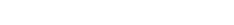### 简介1、 问题理解

2、 移动平均

3、线性回归

4、 K-近邻

5、 自动ARIMA

6、 先知（Prophet）

7、 长短时记忆网络（LSTM）

## 1、问题理解

```#import packages
import pandas as pd
import numpy as np

#to plot within notebook
import matplotlib.pyplot as plt
%matplotlib inline

#setting figure size
from matplotlib.pylab import rcParams
rcParams['figure.figsize'] = 20,10

#for normalizing data
from sklearn.preprocessing import MinMaxScaler
scaler = MinMaxScaler(feature_range=(0, 1))```

```#read the file```#setting index as date
df['Date'] = pd.to_datetime(df.Date,format='%Y-%m-%d')
df.index = df['Date']

#plot
plt.figure(figsize=(16,8))
plt.plot(df['Close'], label='Close Price history')```## 2、移动平均

#### 简介

“平均数”是我们日常生活中最常用的统计量之一。例如，计算平均分数来确定整体表现，或者根据过去几天的平均温度来了解今天的温度——这些都是我们经常做的日常工作。因此，使用这个方法开始用数据集进行预测是个不错的选择。#### 实现

```#creating dataframe with date and the target variable
data = df.sort_index(ascending=True, axis=0)
new_data = pd.DataFrame(index=range(0,len(df)),columns=['Date', 'Close'])
```

```for i in range(0,len(data)):
new_data['Date'][i] = data['Date'][i]
new_data['Close'][i] = data['Close'][i]```

```#splitting into train and validation
train = new_data[:987]
valid = new_data[987:]```

```new_data.shape, train.shape, valid.shape
((1235, 2), (987, 2), (248, 2))```

```train['Date'].min(), train['Date'].max(), valid['Date'].min(), valid['Date'].max()

(Timestamp('2013-10-08 00:00:00'),
Timestamp('2017-10-06 00:00:00'),
Timestamp('2017-10-09 00:00:00'),
Timestamp('2018-10-08 00:00:00'))```

```#make predictions
preds = []
for i in range(0,248):
a = train['Close'][len(train)-248+i:].sum() + sum(preds)
b = a/248
preds.append(b)```

#### 结果

```#calculate rmse
rms=np.sqrt(np.mean(np.power((np.array(valid['Close'])-preds),2)))
rms

104.51415465984348```

```#plot
valid['Predictions'] = 0
valid['Predictions'] = preds
plt.plot(train['Close'])
plt.plot(valid[['Close', 'Predictions']])```#### 推论

RMSE值接近105，但是结果不是很理想(从图中可以看出)。预测值与训练集的观测值的范围相同(开始有上升趋势，然后缓慢下降)。

## 3、线性回归

#### 简介#### 实现

```#setting index as date values
df['Date'] = pd.to_datetime(df.Date,format='%Y-%m-%d')
df.index = df['Date']

#sorting
data = df.sort_index(ascending=True, axis=0)

#creating a separate dataset
new_data = pd.DataFrame(index=range(0,len(df)),columns=['Date', 'Close'])

for i in range(0,len(data)):
new_data['Date'][i] = data['Date'][i]
new_data['Close'][i] = data['Close'][i]

#create features
new_data.drop('Elapsed', axis=1, inplace=True)  #elapsed will be the time stamp```

‘Year’, ‘Month’, ‘Week’, ‘Day’, ‘Dayofweek’, ‘Dayofyear’, ‘Is_month_end’, ‘Is_month_start’, ‘Is_quarter_end’, ‘Is_quarter_start’,  ‘Is_year_end’, and  ‘Is_year_start’.

```new_data['mon_fri'] = 0
for i in range(0,len(new_data)):
if (new_data['Dayofweek'][i] == 0 or new_data['Dayofweek'][i] == 4):
new_data['mon_fri'][i] = 1
else:
new_data['mon_fri'][i] = 0```

```#split into train and validation
train = new_data[:987]
valid = new_data[987:]

x_train = train.drop('Close', axis=1)
y_train = train['Close']
x_valid = valid.drop('Close', axis=1)
y_valid = valid['Close']

#implement linear regression
from sklearn.linear_model import LinearRegression
model = LinearRegression()
model.fit(x_train,y_train)```

#### 结果

```#make predictions and find the rmse
preds = model.predict(x_valid)
rms=np.sqrt(np.mean(np.power((np.array(y_valid)-np.array(preds)),2)))
rms

121.16291596523156```

RMSE值高于之前的方法，这清楚地表明线性回归的表现很差。让我们看看这个图，并理解为什幺线性回归预测效果不是很好:

```#plot
valid['Predictions'] = 0
valid['Predictions'] = preds

valid.index = new_data[987:].index
train.index = new_data[:987].index

plt.plot(train['Close'])
plt.plot(valid[['Close', 'Predictions']])```## 4、k-近邻

#### 简介k近邻介绍:简介

#### 实现

```#importing libraries
from sklearn import neighbors
from sklearn.model_selection import GridSearchCV
from sklearn.preprocessing import MinMaxScaler
scaler = MinMaxScaler(feature_range=(0, 1))```

```#scaling data
x_train_scaled = scaler.fit_transform(x_train)
x_train = pd.DataFrame(x_train_scaled)
x_valid_scaled = scaler.fit_transform(x_valid)
x_valid = pd.DataFrame(x_valid_scaled)

#using gridsearch to find the best parameter
params = {'n_neighbors':[2,3,4,5,6,7,8,9]}
knn = neighbors.KNeighborsRegressor()

model = GridSearchCV(knn, params, cv=5)

#fit the model and make predictions
model.fit(x_train,y_train)
preds = model.predict(x_valid)```

#### 结果

```#rmse
rms=np.sqrt(np.mean(np.power((np.array(y_valid)-np.array(preds)),2)))
rms

115.17086550026721```

RMSE值并没有太大的差异，但是一个预测值和实际值的曲线图应该提供一个更清晰的理解。

```#plot
valid['Predictions'] = 0
valid['Predictions'] = preds
plt.plot(valid[['Close', 'Predictions']])
plt.plot(train['Close'])```#### 推论

RMSE值与线性回归模型近似，图中呈现出相同的模式。与线性回归一样，kNN也发现了2018年1月的下降，因为这是过去几年的模式。我们可以有把握地说，回归算法在这个数据集上表现得并不好。

## 5、自动ARIMA

#### 简介

ARIMA是一种非常流行的时间序列预测统计方法。ARIMA模型使用过去的值来预测未来的值。ARIMA中有三个重要参数：

p(用来预测下一个值的过去值)

q(用来预测未来值的过去预测误差)

d(差分的顺序)

ARIMA的参数优化需要大量时间。因此我们将使用自动 ARIMA，自动选择误差最小的(p,q,d)最佳组合。要了解更多关于自动ARIMA的工作原理，请参阅本文：

#### 实现

```from pyramid.arima import auto_arima

data = df.sort_index(ascending=True, axis=0)

train = data[:987]
valid = data[987:]

training = train['Close']
validation = valid['Close']

model = auto_arima(training, start_p=1, start_q=1,max_p=3, max_q=3, m=12,start_P=0, seasonal=True,d=1, D=1, trace=True,error_action='ignore',suppress_warnings=True)
model.fit(training)

forecast = model.predict(n_periods=248)
forecast = pd.DataFrame(forecast,index = valid.index,columns=['Prediction'])```

#### 结果

```rms=np.sqrt(np.mean(np.power((np.array(valid['Close'])-np.array(forecast['Prediction'])),2)))
rms
44.954584993246954
#plot
plt.plot(train['Close'])
plt.plot(valid['Close'])
plt.plot(forecast['Prediction'])```## 6、先知（Prophet）

#### 实现

```#importing prophet
from fbprophet import Prophet

#creating dataframe
new_data = pd.DataFrame(index=range(0,len(df)),columns=['Date', 'Close'])

for i in range(0,len(data)):
new_data['Date'][i] = data['Date'][i]
new_data['Close'][i] = data['Close'][i]

new_data['Date'] = pd.to_datetime(new_data.Date,format='%Y-%m-%d')
new_data.index = new_data['Date']

#preparing data
new_data.rename(columns={'Close': 'y', 'Date': 'ds'}, inplace=True)

#train and validation
train = new_data[:987]
valid = new_data[987:]

#fit the model
model = Prophet()
model.fit(train)

#predictions
close_prices = model.make_future_dataframe(periods=len(valid))
forecast = model.predict(close_prices)```

#### 结果

```#rmse
forecast_valid = forecast['yhat'][987:]
rms=np.sqrt(np.mean(np.power((np.array(valid['y'])-np.array(forecast_valid)),2)))
rms

57.494461930575149

#plot
valid['Predictions'] = 0
valid['Predictions'] = forecast_valid.values

plt.plot(train['y'])
plt.plot(valid[['y', 'Predictions']])```## 7、长短期记忆网络(LSTM)

#### 简介

LSTM 算法广泛应用于序列预测问题中，并被证明是一种非常有效的方法。它们之所表现如此出色，是因为LSTM能够存储重要的既往信息，并忽略不重要的信息。

LSTM有三个门：

#### 实现

#importing required libraries

from sklearn.preprocessing import MinMaxScaler

from keras.models import Sequential

from keras.layers import Dense,Dropout, LSTM

#creating dataframe

data = df.sort_index(ascending=True, axis=0)

new_data = pd.DataFrame(index=range(0,len(df)),columns=[‘Date’, ‘Close’])

for i in range(0,len(data)):

new_data[‘Date’][i] = data[‘Date’][i]

new_data[‘Close’][i] = data[‘Close’][i]

#setting index

new_data.index = new_data.Date

new_data.drop(‘Date’, axis=1, inplace=True)

```
#creating train and test sets
dataset = new_data.values

train = dataset[0:987,:]
valid = dataset[987:,:]

#converting dataset into x_train and y_train
scaler = MinMaxScaler(feature_range=(0, 1))```

```scaled_data = scaler.fit_transform(dataset)

x_train, y_train = [], []
for i in range(60,len(train)):
x_train.append(scaled_data[i-60:i,0])
y_train.append(scaled_data[i,0])
x_train, y_train = np.array(x_train), np.array(y_train)

x_train = np.reshape(x_train, (x_train.shape,x_train.shape,1))

# create and fit the LSTM network```

```model = Sequential()

model.fit(x_train, y_train, epochs=1, batch_size=1, verbose=2)
```

```#predicting 246 values, using past 60 from the train data
inputs = new_data[len(new_data) - len(valid) - 60:].values
inputs = inputs.reshape(-1,1)
inputs  = scaler.transform(inputs)

X_test = []
for i in range(60,inputs.shape):
X_test.append(inputs[i-60:i,0])
X_test = np.array(X_test)

X_test = np.reshape(X_test, (X_test.shape,X_test.shape,1))
closing_price = model.predict(X_test)
closing_price = scaler.inverse_transform(closing_price)```

#### 结果

```rms=np.sqrt(np.mean(np.power((valid-closing_price),2)))
rms

11.772259608962642```

```#for plotting
train = new_data[:987]
valid = new_data[987:]
valid['Predictions'] = closing_price
plt.plot(train['Close'])
plt.plot(valid[['Close','Predictions']])```